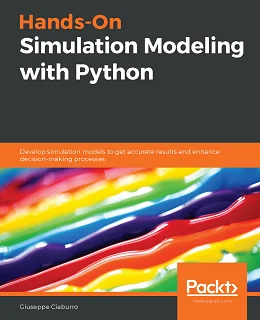# Hands-On Simulation Modeling with Python### eBook Details:

• Paperback: 346 pages
• Publisher: WOW! eBook (July 17, 2020)
• Language: English
• ISBN-10: 1838985093
• ISBN-13: 978-1838985097

### eBook Description:

Hands-On Simulation Modeling with Python: A comprehensive guide to enhancing your simulation modeling skills by creating and analyzing digital prototypes of a physical model using Python

Simulation modeling helps you to create digital prototypes of physical models to analyze how they work and predict their performance in the real world. With this comprehensive guide, you’ll understand various computational statistical simulations using Python.

Starting with the fundamentals of simulation modeling, you’ll understand concepts such as randomness and explore data generating processes, resampling methods, and bootstrapping techniques. You’ll then cover key algorithms such as Monte Carlo simulations and Markov decision processes, which are used to develop numerical simulation models, and discover how they can be used to solve real-world problems. As you advance, you’ll develop simulation models to help you get accurate results and enhance decision-making processes. Using optimization techniques, you’ll learn to modify the performance of a model to improve results and make optimal use of resources. The book will guide you in creating a digital prototype using practical use cases for financial engineering, prototyping project management to improve planning, and simulating physical phenomena using neural networks.

• Gain an overview of the different types of simulation models
• Get to grips with the concepts of randomness and data generation process
• Understand how to work with discrete and continuous distributions
• Work with Monte Carlo simulations to calculate a definite integral
• Find out how to simulate random walks using Markov chains
• Obtain robust estimates of confidence intervals and standard errors of population parameters
• Discover how to use optimization methods in real-life applications
• Run efficient simulations to analyze real-world systems

By the end of this Hands-On Simulation Modeling with Python book, you’ll have learned how to construct and deploy simulation models of your own to overcome real-world challenges.